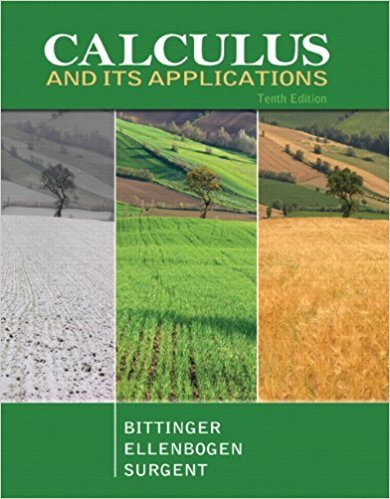×
×

# Solutions for Chapter R.5: Nonlinear Functions and Models## Full solutions for Calculus and Its Applications | 10th Edition

ISBN: 9780321694331Solutions for Chapter R.5: Nonlinear Functions and Models

Solutions for Chapter R.5
4 5 0 400 Reviews
31
1
##### ISBN: 9780321694331

Chapter R.5: Nonlinear Functions and Models includes 113 full step-by-step solutions. Since 113 problems in chapter R.5: Nonlinear Functions and Models have been answered, more than 26327 students have viewed full step-by-step solutions from this chapter. This textbook survival guide was created for the textbook: Calculus and Its Applications, edition: 10. Calculus and Its Applications was written by and is associated to the ISBN: 9780321694331. This expansive textbook survival guide covers the following chapters and their solutions.

Key Calculus Terms and definitions covered in this textbook
• Absolute value of a vector

See Magnitude of a vector.

• Bar chart

A rectangular graphical display of categorical data.

• Bounded

A function is bounded if there are numbers b and B such that b ? ƒ(x) ? B for all x in the domain of f.

• DMS measure

The measure of an angle in degrees, minutes, and seconds

• End behavior asymptote of a rational function

A polynomial that the function approaches as.

• First quartile

See Quartile.

• Graph of parametric equations

The set of all points in the coordinate plane corresponding to the ordered pairs determined by the parametric equations.

• Implied domain

The domain of a function’s algebraic expression.

• Multiplication property of equality

If u = v and w = z, then uw = vz

• Multiplicative identity for matrices

See Identity matrix

• Polynomial interpolation

The process of fitting a polynomial of degree n to (n + 1) points.

• Positive linear correlation

See Linear correlation.

• Projectile motion

The movement of an object that is subject only to the force of gravity

• Randomization

The principle of experimental design that makes it possible to use the laws of probability when making inferences.

• Remainder polynomial

See Division algorithm for polynomials.

• Richter scale

A logarithmic scale used in measuring the intensity of an earthquake.

• Sine

The function y = sin x.

• Square matrix

A matrix whose number of rows equals the number of columns.

• Subtraction

a - b = a + (-b)

• Upper bound test for real zeros

A test for finding an upper bound for the real zeros of a polynomial.

×